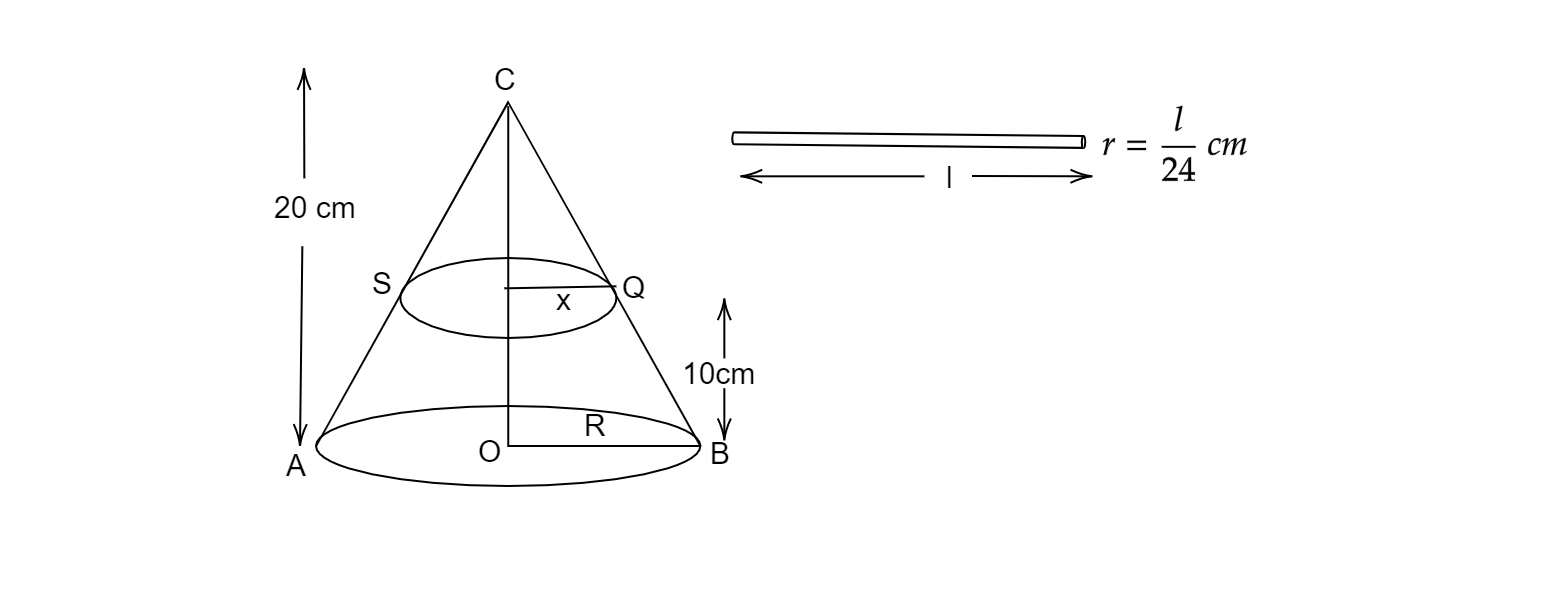# A solid metallic right circular cone 20 cm high and whose vertical angle is $60^{o}$, is cut into two parts at the middle of its height by a plane parallel to it base.If the frustum so obtained drawn into a wire of diameter $\frac{1}{12}$cm, find the length of the wire.

Given: A solid metallic right circular cone 20 cm high and whose vertical angle is $60^{o}$, is cut into two parts at the middle of its height by a plane parallel to it base. If the frustum so obtained drawn into a wire of diameter $\frac{1}{12}$cm.

To do: To find the length of the wire.

Solution:Let ACB be the cone whose vertical angle $\angle ACB = 60^{o}$.

Let $R$ and $x$ be the radii of the lower and upper end of the frustum.

Here, height of the cone, $OC = 20 cm=H$

Height $CP = h = 10\ cm$

Let us consider P as the mid-Point of OC

Cutting the cone into two parts through P.

OP =$\frac{20}{2}= 10\ cm$

Also,$\angle ACO$ and $\angle OCB =$\frac{1}{2} \times 60^{o} =30^{o} $After cutting cone CQS from cone CBA, the remaining solid obtained is a frustum. Now, in triangle CPQ$tan30^{o}=\frac{x}{10}\frac{1}{\sqrt{3}} =\frac{x}{10}\Rightarrow x=\frac{10}{\sqrt{3}}\ cm$In triangle COB$tan30^{o}=\frac{R}{20}\Rightarrow \frac{1}{\sqrt{3}} =\frac{R}{20}\Rightarrow R=\frac{20}{\sqrt{3}}$Volume of the frustum,$V=\frac{1}{3} \pi \left( R^{2} H-x^{2} h\right)\Rightarrow V=\frac{1}{3} \pi \left(\left(\frac{20}{\sqrt{3}}\right)^{2} .20-\left(\frac{10}{\sqrt{3}}\right)^{2} .10\right)\Rightarrow V=\frac{1}{3} \pi \left(\frac{400\times 20}{3} -\frac{100\times 10}{3}\right)\Rightarrow V=\frac{1}{3} \pi \left(\frac{8000-1000}{3}\right)\Rightarrow V=\frac{7000}{9} \pi \ cm^{3}$Let us assume the length of the wire l. Given diameter of the wire obtained from the frustum$=\frac{1}{12}\ cm$Radius of the wire,$r=\frac{1}{2} \times \frac{1}{12} =\frac{1}{24}\ cm$Volume of the wire$=\pi r^{2} l=\pi \left(\frac{1}{24}\right)^{2} l=\frac{\pi l}{576} cm^{3}$The volumes of the frustum and the wire formed are equal,$\frac{7000}{9} \pi =\frac{\pi l}{576}\Rightarrow \frac{7000}{9} =\frac{l}{576}\Rightarrow l=\frac{7000\times 576}{9}\Rightarrow l=448000\ cm\Rightarrow l=4480\ cm\$

Therefore, length of the wire is 480 cm.

Updated on: 10-Oct-2022

33 Views